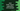# Static import in Java explanation with example## Static import in Java explanation with example :

Using static import in Java, we can access any public static member of a class directly without using its class name. For example, to find the square root of a number , we have sqrt() method defined in Math class. Since it is a public static class (public static double sqrt(double a)), we can call it directly using the class name like Math.sqrt(4). But , we can also use static import of the class Math and call sqrt method directly like sqrt(4).

Imagine a large class with thousand lines of code and we are using static methods like sqrt in each line. In that case, using static import will save us a lot of time as we don’t need to type the same class name again and again.

### Java example program without using static import :

``````class Main{
public static void main(String args[]){
System.out.println("The value of PI is : "+Math.PI);
System.out.println("Square root of 16 is : "+Math.sqrt(16));
}
}``````

It will produce the following output :

``````The value of PI is : 3.141592653589793
Square root of 16 is : 4.0``````

Now, Let’s see how to use static import on this program

### Java example program using static import :

``````import static java.lang.Math.*;
import static java.lang.System.out;

class Main{
public static void main(String args[]){
out.println("The value of PI is : "+PI);
out.println("Square root of 16 is : "+sqrt(16));
}
}``````

This program will also print the same output as above. Only difference is that we have used two imports (static imports) in the beginning, so System.out.println() is written as out.println() and Math.PI,Math.sqrt() are written as PI,sqrt() respectively.

### Ambiguity :

If two static imports have members with same name, it will throw an error. Because, it will not be able to determine which member to select in the absence of class name.

``````import static java.lang.Integer.*;

class Main{
public static void main(String args[]){
System.out.println(MAX_VALUE);
}
}``````

This program will work . But :

``````import static java.lang.Integer.*;
import static java.lang.Long.*;

class Main{
public static void main(String args[]){
System.out.println(MAX_VALUE);
}
}``````

It will throw one compiler error stating as reference to MAXVALUE is ambiguous._ because MAXVALUE_ is present in both of the imported packages.

### Drawbacks of Static import :

Use static import if your program needs frequent access to static members of a different class. But importing all static members from a class may harm the readability of the program. Because it is hard to find which class contains a value only by reading its name. Use it in your code , but make sure that it is understandable to other peoples as well.